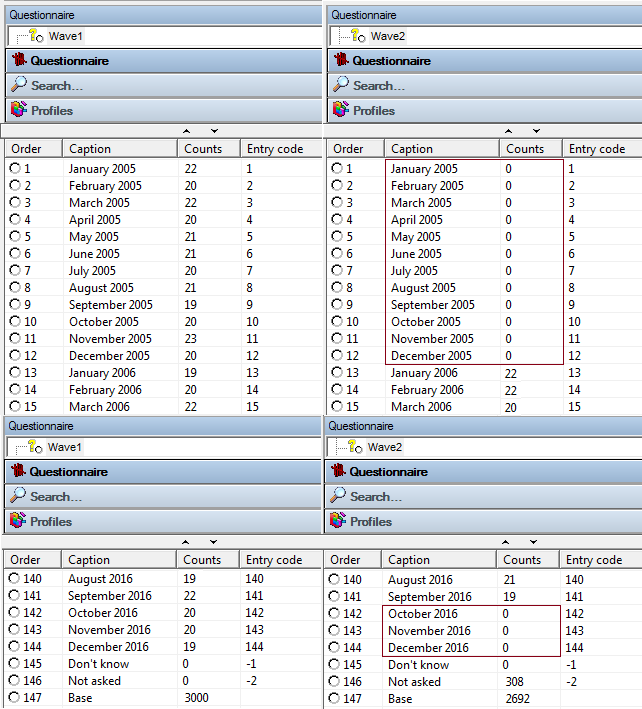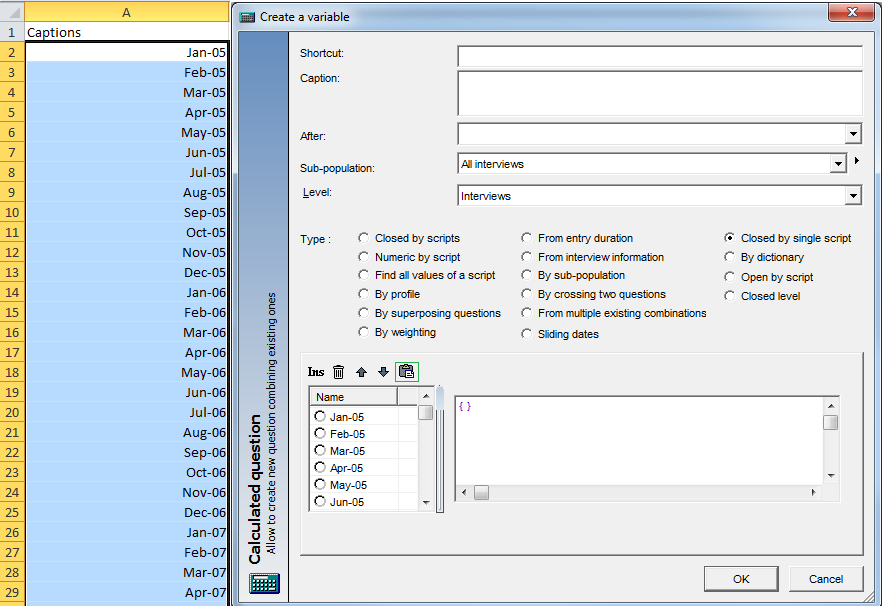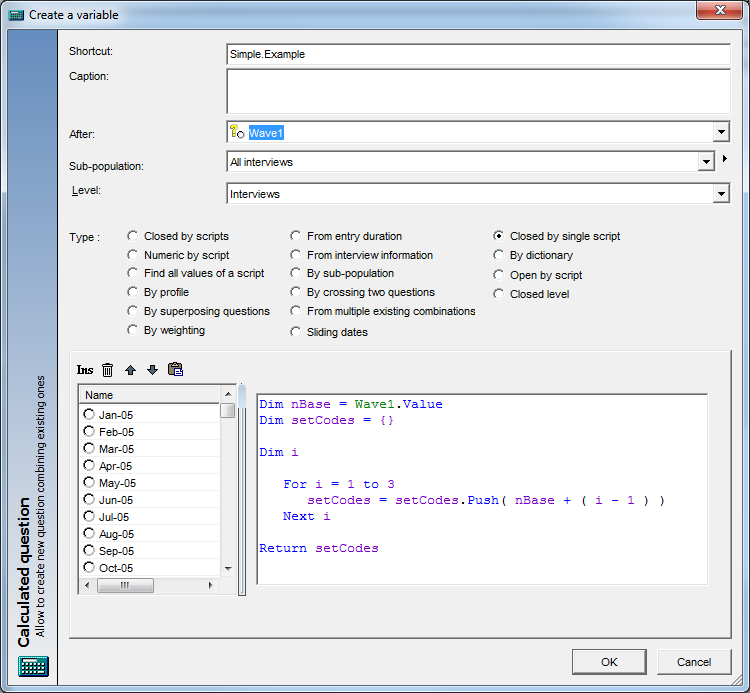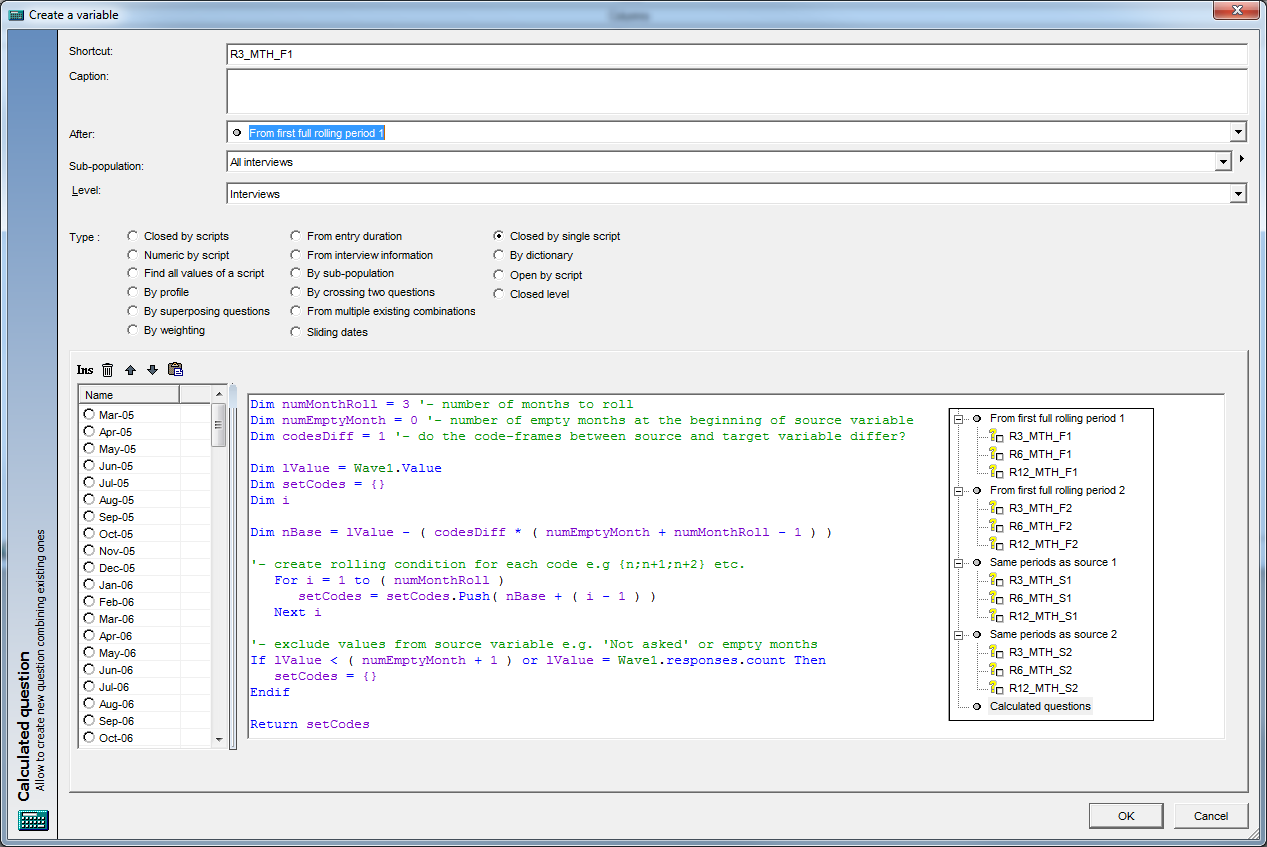Scroll

# Rolling Wave Periods Made Easy

Follow
 Summary If you have your waves in a coded variable, it can be tedious or time consuming to create a rolling time period variable, especially if you have a large number of waves. This article shows how to create a rolling time period variable using a single script. It's an alternative to the 'Group responses' or 'Closed by scripts' methods. to Askia Analyse. Written for Data Processors, Analysts, Researchers, Statistical Analysts. Keywords Closed by single script, Calculated variable, Wave, Period, Rolling, Month, Quarter, Year, Group responses, Closed by single script, Code frame, Sliding dates.

When you open it you will see two variables in the questionnaire tree and several calculated questions. The two variables are typical examples of wave variables you might have in one of your data files. Wave1 has data in all its codes whereas Wave2 has data in all but its first 12 and last 3 codes.With a 144 codes in each variable you can imagine that creating all the required rolling time periods will become quite a chore - the links below show this for two methods:

However, for repetitive tasks, Analyse scripts nearly always provide a quicker solution. This time we use aggregated scripts to write one piece of syntax that takes care of all the re-coding in one go.

Start by creating a closed by single script variable. Then, paste in your code-frame using the clipboard icon highlighted in the screenshot below:Now let's go through the simplest form of syntax required where you have the same number of codes (144) in the target question and each of its codes are rolled 3 months from the source question:The syntax of simply Wave1.Value would return the value of each each code from Wave1 into the equivalent code of Simple.Example (See variable: Simple.Ex1). In order to return the 3 months rolling data, for every value of Wave1 we need to set it to that and the next two values in Simple.Example. e.g. code 1 (January 2005) in Wave1 will need to return codes {1;2;3} in Simple.Example, this way it counts towards {January 2005; February 2005; March 2005} in the resultant variable.

So this is why in the above syntax we have set a for loop of 3 iterations. The Push keyword will add to the array, setCodes, on each iteration:

i = 1, setCodes = {Wave1.Value + 0}

i = 2, setCodes = {Wave1.Value + 0; Wave1.Value + 1}

i = 3, setCodes = {Wave1.Value + 0; Wave1.Value + 1; Wave1.Value + 2}

As mentioned, this is the simple form of the syntax. We can make it a bit more flexible by adding a few parameters. The above will suffice in most cases but the following is useful reference for dealing with things like your target code-frame starting at the first full n month period, dealing with empty waves and excluding certain other codes.The parameters to change are the first three lines in the syntax above and the calculated variables in the chapters show how these are apply to different rolling variable scenarios.

Don't forget, managing banding of actual date variables is also made easy using the 'Sliding dates' calculated variable type. The above explanation is for dealing with coded wave variables as a start point.